# About Digital to Analog Converter (DAC) and Its Applications

Why we need data converters? In the real world, most data are available in the form of analog in nature. We have two types of converters analog to digital converter and digital to analog converter. While manipulating the data, these two converting interfaces are essential to digital electronic equipment and an analog electric device which to be processed by a processor in order produce required operation.

For example, take the below DSP illustration, an ADC converts the analog data collected by audio input equipment such as a microphone (sensor), into a digital signal that can be processed by a computer. The computer may add sound effects. Now a DAC will process the digital sound signal back into the analog signal that is used by audio output equipment such as a speaker.

## Digital to Analog Converter (DAC)

Digital to Analog Converter (DAC) is a device that transforms digital data into an analog signal. According to the Nyquist-Shannon sampling theorem, any sampled data can be reconstructed perfectly with bandwidth and Nyquist criteria.

A DAC can reconstruct sampled data into an analog signal with precision. The digital data may be produced from a microprocessor, Application Specific Integrated Circuit (ASIC), or Field Programmable Gate Array (FPGA), but ultimately the data requires the conversion to an analog signal in order to interact with the real world.

### D/A Converter Architectures

There are two methods commonly used for digital to analog conversion: Weighted Resistors method and the other one is using the R-2R ladder network method.

### DAC using Weighted Resistors method

The below shown schematic diagram is DAC using weighted resistors. The basic operation of DAC is the ability to add inputs that will ultimately correspond to the contributions of the various bits of the digital input. In the voltage domain, that is if the input signals are voltages, the addition of the binary bits can be achieved using the inverting summing amplifier shown in the below figure.

In the voltage domain, that is if the input signals are voltages, the addition of the binary bits can be achieved using the inverting summing amplifier shown in the above figure.

The input resistors of the op-amp have their resistance values weighted in a binary format. When the receiving binary 1 the switch connects the resistor to the reference voltage. When the logic circuit receives binary 0, the switch connects the resistor to ground. All the digital input bits are simultaneously applied to the DAC.

The DAC generates analog output voltage corresponding to the given digital data signal. For the DAC the given digital voltage is b3 b2 b1 b0 where each bit is a binary value (0 or 1). The output voltage produced at output side is

V0=R0/R (b3+b2/2+b1/4+b0/8) Vref

As the number of bits is increasing in the digital input voltage, the range of the resistor values becomes large and accordingly, the accuracy becomes poor.

### R-2R Ladder Digital to Analog Converter (DAC)

The R-2R ladder DAC constructed as a binary-weighted DAC that uses a repeating cascaded structure of resistor values R and 2R. This improves the precision due to the relative ease of producing equal valued-matched resistors (or current sources).

The above figure shows the 4-bit R-2R ladder DAC. In order to achieve high-level accuracy, we have chosen the resistor values as R and 2R. Let the binary value B3 B2 B1 B0, if b3=1, b2=b1=b0=0, then the circuit is shown in the figure below it is a simplified form of the above DAC circuit. The output voltage is V0=3R(i3/2)= Vref/2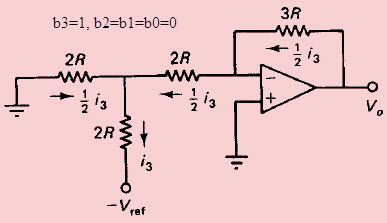Similarly, If b2=1, and b3=b1=b0=0, then the output voltage is V0=3R(i2/4)=Vref/4 and the circuit is simplified as below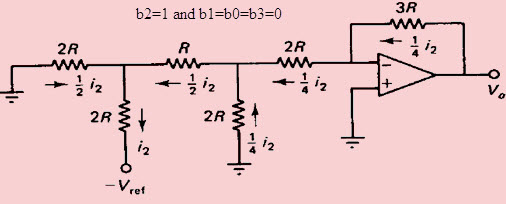If b1=1 and b2=b3=b0=0, then the circuit shown in the figure below it is a simplified form of the above DAC circuit. The output voltage is V0=3R(i1/8)= Vref/8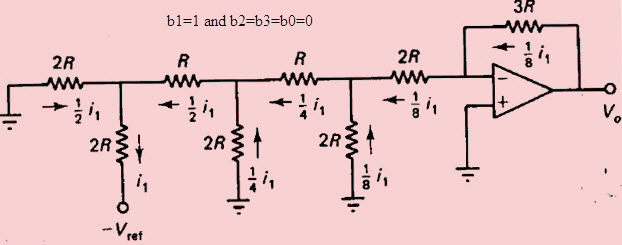Finally, the circuit is shown in below corresponding to the case where b0=1 and b2=b3=b1=0. The output voltage is V0=3R(i0/16) = Vref/16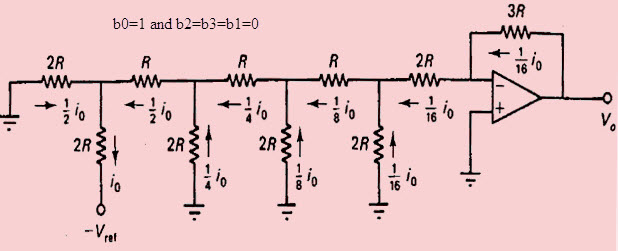In this way, we can find that when the input data is b3b2b1b0 (where individual bits are either 0 or 1), then the output voltage is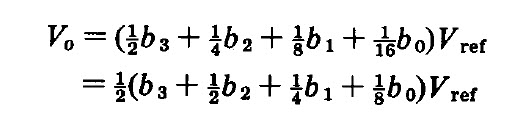#### Applications of Digital to Analog Converter

DACs are used in many digital signal processing applications and many more applications. Some of the important applications are discussed below.

#### Audio Amplifier

DACs are used to produce DC voltage gain with Microcontroller commands. Often, the DAC will be incorporated into an entire audio codec which includes signal processing features.

#### Video Encoder

The video encoder system will process a video signal and send digital signals to a variety of DACs to produce analog video signals of various formats, along with optimizing of output levels. As with audio codecs, these ICs may have integrated DACs.

#### Display Electronics

The graphic controller will typically use a lookup table to generate data signals sent to a video DAC for analog outputs such as Red, Green, Blue (RGB) signals to drive a display.

#### Data Acquisition Systems

Data to be measured is digitized by an Analog-to-Digital Converter (ADC) and then sent to a processor. The data acquisition will also include a process control end, in which the processor sends feedback data to a DAC for converting to analog signals.

#### Calibration

The DAC provides dynamic calibration for gain and voltage offset for accuracy in test and measurement systems.

#### Motor Control

Many motor controls require voltage control signals, and a DAC is ideal for this application which may be driven by a processor or controller.

#### Data Distribution System

Many industrial and factory lines require multiple programmable voltage sources, and this can be generated by a bank of DACs that are multiplexed. The use of a DAC allows the dynamic change of voltages during operation of a system.

#### Digital Potentiometer

Almost all digital potentiometers are based on the string DAC architecture. With some reorganization of the resistor/switch array, and the addition of an I2C compatible interface, a fully digital potentiometer can be implemented.